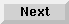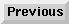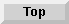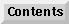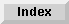Eloquence Language Manual

# 10 Matrix Operations

This chapter describes the operations which can be performed on entire arrays. Some of the operations are used exclusively with matrix or vector arrays.

A matrix is a two-dimensional array. A vector is a one-dimensional array. Matrices and vectors are defined the same as other arrays.

These matrix statements and functions are described in this chapter:

Copy values to the array.
MAT PRINT and MAT PRINT#
Write values from the array.
MAT INPUT, MAT . . . CON, MAT . . . ZER and MAT-initialize
Assign values to the array.
MAT
Arithmetic operations are performed on the entire array.
SUM
Returns the sum of all elements of the array.
ROW
Returns the number of rows in the array.
COL
Returns the number of columns in the array.
MAT . . . CSUM
Finds the sum of all columns of the matrix.
MAT . . . RSUM
Finds the sum of all rows of the matrix.
Chapter contents:

Redimensioning Arrays